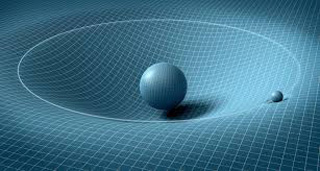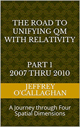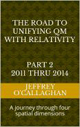# Does Dark energy violate the conservations laws?

Dark Energy is a repulsive force that is the dominant component (69.4 percent) of the universe. The remaining portion consists of ordinary matter and dark matter. Dark Energy, in contrast to both forms of matter, is relatively uniform in time and space and is gravitationally repulsive, not attractive, within the volume it occupies. The nature of Dark Energy is still not well understood.However if you have a Universe full of stuff it’s virtually impossible to keep it static. The fabric of our Universe, at least in General Relativity, must either expand or contract on the largest scales. But if you have a Universe filled with Dark Energy, as we appear to have, something even more troubling happens: the total amount of energy contained within our observable Universe increases over time, with no end in sight. Doesn’t this violate the conservation of energy?

That depends on what is responsible for that energy.

Einstein was aware, while working on is General Theory of Relativity that it is virtually impossible to keep a Universe full of stuff static so 1917, he added a cosmological constant to his equations.  Some believe this would provide one of simplest mathematical explanations for Dark Energy however it is difficult for many to conceptually integrate it with the physical imagery that is provided by his theory.

For example, in Einstein’s General Theory of Relativity he derived gravity in terms of a curvature in a space-time metric.  One can form a relatively simple physical image of it based on how objects such as a ball is accelerated on a curved two dimensional surface on the earth and then extrapolating that to a curvature in a space-time metric.

Yet one of the difficulties some may have in forming a similar image of Dark Energy is because Einstein chose to define gravity in terms of time or a space-time dimension while the expansion of Dark Energy appears to be related to the spatial not the time properties of our universe.

However he did provide a way to understand the spatial expansion associated with it in terms of physical image similar to the one he provided for gravity while at the same time answering the question razed earlier if it violated the conservation laws of physics.

He gave us the ability to do this when he derived gravitational energy in a space-time environment in terms of time and the constant velocity of light because that provided a method of converting a unit of time a space-time environment with unit of space in four *spatial* dimensions.   Additionally because the velocity of light is universally constant he also defined a one to one quantitative correspondence between his space-time universe and one made up of four *spatial* dimensions.

This fact that one can use Einstein’s theories to qualitatively and quantitatively derive the spatial properties of energy in a space-time universe in terms of four *spatial* dimensions is one the bases of assuming as was done in the article “Defining energy” Nov 27, 2007 that all forms of energy can be derived in terms of a spatial displacement in a "surface" of a three-dimensional space manifold with respect to a fourth *spatial* dimension.

In other words one can not only use Einstein’s equations to quantitatively and qualitatively derive how energy interacts with time in a space-time dimension but also how it would interact with its spatial equivalent in four *spatial* dimensions.

The study of thermodynamics tells us those particles with high temperature or energy move or flow to areas of low energy very similar to how water flows form an elevated or "high energy" point to a lower one.

However the fact that Einstein defines energy in terms of field properties of space-time means that one must also consider the thermodynamics of those fields.  In other words because he defined energy in terms of a dimensional properties of space we must assume even with no particle matter present that it contains energy.  In other words one must apply the concept of thermodynamics to those field properties.

For example if the walls of an above ground pool filled with water collapse the elevated two-dimensional surface of the water will flow or expand and accelerate outward towards the three-dimensional environment sounding it.

Yet we know from observations of the cosmic background radiation that presently our three-dimensional universe has an average energy component equal to about 3.7 degrees Kelvin.

However this means that according to concepts developed in the article “Defining energy" (mentioned earlier) the three-dimensional "surface" of our universe which has an average energy component of 3.7 degree Kelvin would be elevated with respect to a fourth *spatial* dimension.

As was mentioned earlier Einstein gave us the ability to define energy in terms of a spatial displacement in a "surface" of a three dimensional space manifold with respect to a forth *spatial* dimension as well as a time dimension.  Therefore, similar to the surface water in a pool if that "surface" was elevated with respect to a fourth *spatial* dimension as Einstein tell us as it must be because, as was just mentioned it has the energy associated 3.7 degree Kelvin then it would accelerate outward for the same reason as the water in a pool whose sides had collapsed.

In other words one can qualitatively understand the casually of the Dark energy  in term of the physical image of water expanding out of a collapsed pool.

However if this theoretical model is correct it means that Dark Energy would not violate the conservation laws because it would be compensated for by the cooling of the universe and not with a physical component of space similar to how energy released from the water in a dam is does violate the conservation laws because it is compensated for by the loss of its potential energy.

In other words the total amount of energy contained within our observable Universe does not increase over time because the energy of its expansion is compensated for by its loss of energy due to its cooling.

It should be noted there is nothing new or revolutionary about this concept because, as was shown above, it is consistent with the Einstein General and special Theories Relativity and all of the currently accepted laws of physics that govern the evolution of the universe.

Yet some may feel that this is an over simplification of what appears on the surface to be a rather complex phenomena such as Dark Energy but is no more simplistic that the one use to help us understand how gravity works in a space-time environment.  Granted the math behind this concept may be complex and difficult to understand as it is with the gravitational curvature in space-time however that does not mean that we cannot use it to understand its causality.

It should be remember that Einstein’s genius and the symmetry of his mathematics allows us to choose whether to define the forces associated with gravity and Dark Energy in either four *spatial* dimensions or four dimensional space-time.

Later Jeff

 The Road to Unifying QM with Relativity part 1 2007 thru 2010Ebook \$8.00Paper Back \$15.00 The Road to Unifying QM with Relativity part 2 2011 thru 2014Ebook \$8.00Paper Back \$16.00 The Road to Unifying QM with Relativity part 3 2015 thru 2020Ebook \$8.00Paper Back \$18.00